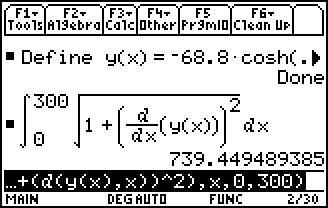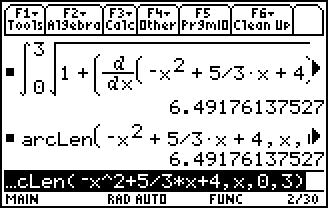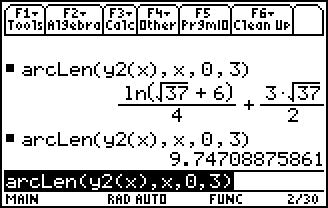# Activities

••• ##### Subject Area

• Math: Calculus: Applications of Integral, Antiderivatives and Definite Integrals

• ##### Author9-12

15 Minutes

• ##### Device
• TI-89 / TI-89 Titanium
• TI-92 Plus / Voyage™ 200
• ##### Report an Issue

Gateway Arc Length#### Activity Overview

Students investigate the arc length of the Gateway Arch. They will use the Pythagorean Theorem to approximate and use Calculus to find the exact solution.

#### Key Steps

•Students will investigate the arc length of the Gateway Arch. They will find the distance that someone would travel if they rode the elevator tram to reach the top of the arch. Students will use the Pythagorean Theorem to approximate and use Calculus to find the exact solution.

•Students will use CAS capabilities (including the arcLen command) to solve a variety of arc length questions. Students will also find the arc length of a parametric equation by hand.

•Students will also answer exam-like questions to help them see what they are expected to know. They will be expected to know the arc length formula and answer multiple-choice questions without a calculator.